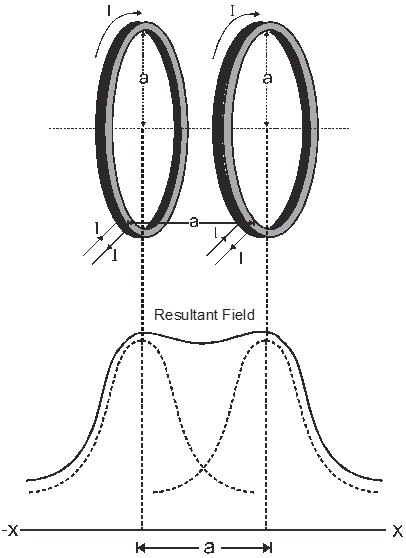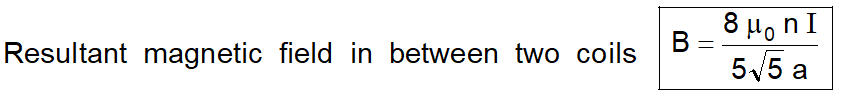Most Affordable JEE | NEET | 8,9,10 Preparation by Kota's Top IITian Doctor Faculties

# Helmholtz Coils | Magnetic Field between two Coils | eSaralHelmholtz coil is named after the German physicist Hermann von Helmholtz. It is comprised of two identical magnetic coils positioned in parallel to each other, and their centers are aligned in the same x-axis. The two coils are separated by a distance equal to the radius like a mirror image as shown in Figure 1. When current is passing through the two coils in the same direction, it generates a uniform magnetic field in a three-dimension region of space within the coils. Helmholtz coils are normally used for scientific experiments, magnetic calibration, to cancel background (earth’s) magnetic field, and for electronic equipment magnetic field susceptibility testing.

## Helmholtz Coils

The two coaxial coils of equal radii placed at a distance equal to the radius of either of the coils and in which the same current in the same direction is flowing are known as Helmholtz coils.

For these coils $x=\frac{a}{2}, I_{1}=I_{2}=I, a_{1}=a_{2}=a$The two coils are placed mutually parallel to each other these coils are used to produce a uniform magnetic field. In between two coils along the axis at the middle point rate of change of magnetic field is constant, so if distance increases from a coil magnetic field decrease, but the distance from another coil decreases, so magnetic field due to second coil increases and hence the resultant magnetic field produced in the region between two coils remains uniform.or $\mathrm{B}=0.76 \frac{\mu_{0} n \mathrm{I}}{a} \quad$ or $\mathrm{B}=1.423 \mathrm{B}_{\mathrm{C}}$

(Bc is a magnetic field at the center of a single coil.)\

Click here for the Video tutorials of Magnetic Effect of Current Class 12